Introduction to Division

 Problem Set 1 Problem Set 2 Problem Set 3Select a problem set using the buttons above, then use your mouse or tab key to select a question. Fill in the blank with the correct answer for each problem. When you have answered all of the questions, ask Charlie how you did.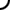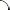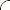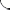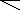Perform each division operation. If there is a nonzero remainder then write it after the quotient separated by the capital letter 'R'. Example: 7 ÷ 2 = 3R1   24 ÷ 6 = 56 ÷ 7 = 25 ÷ 4 = 54 ÷ 7 = 121 ÷ 9 = 152 ÷ 13 =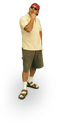Perform each division operation. If there is a nonzero remainder then write it after the quotient separated by the capital letter 'R'. Example: 7 ÷ 2 = 3R1   72 ÷ 9 = 42 ÷ 7 = 17 ÷ 3 = 57 ÷ 2 = 250 ÷ 11 = 427 ÷ 12 =Perform each division operation. If there is a nonzero remainder then write it after the quotient separated by the capital letter 'R'. Example: 7 ÷ 2 = 3R1   63 ÷ 3 = 32 ÷ 8 = 33 ÷ 5 = 19 ÷ 7 = 236 ÷ 10 = 362 ÷ 12 =©  2009  LarryPerezWeb Design:  Patrick Quigley